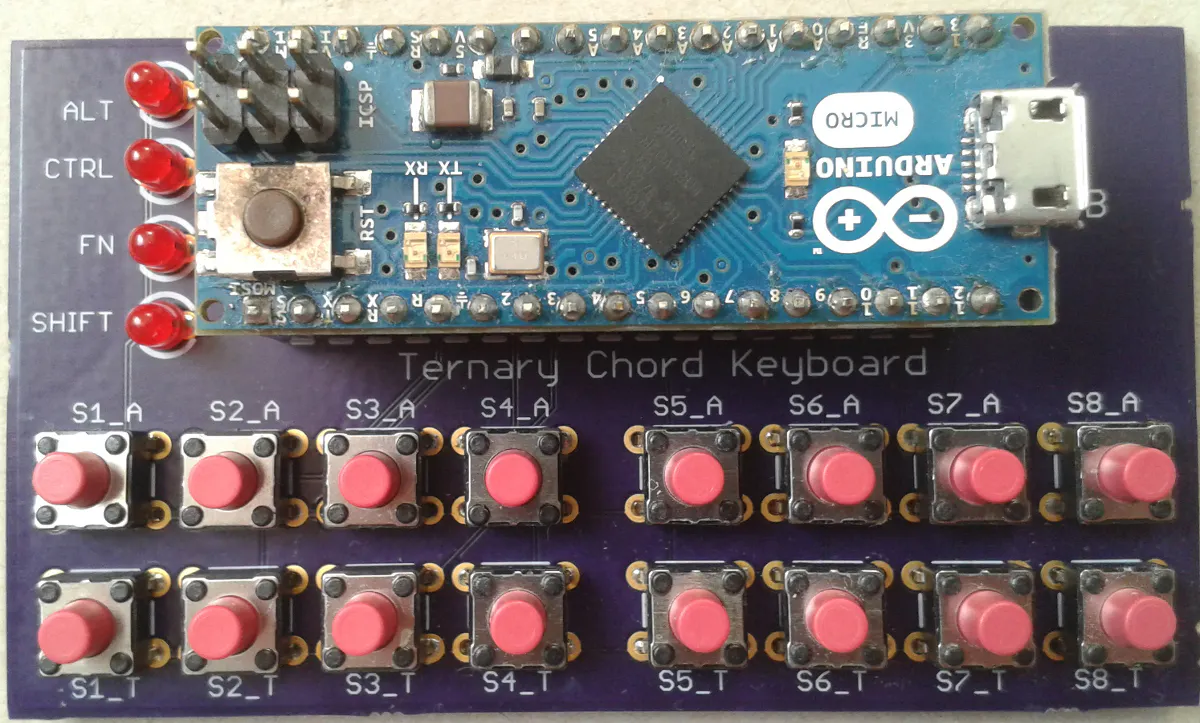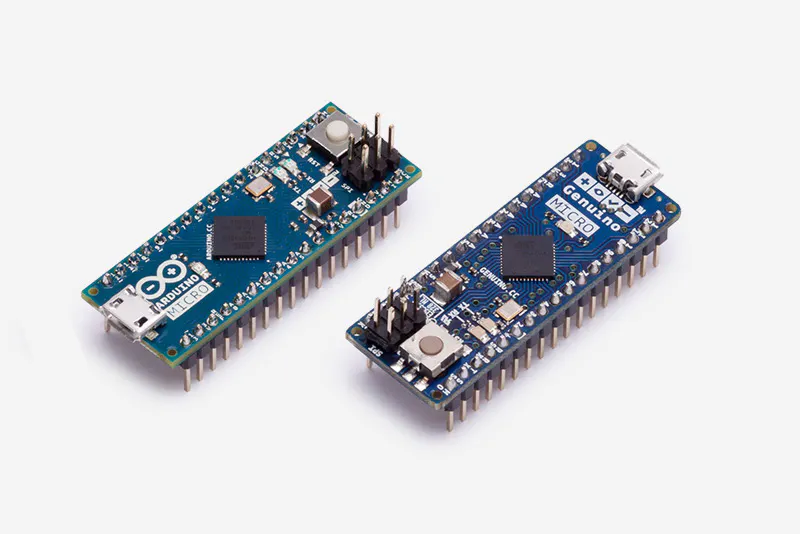# Ternary Chord Keyboard

Accukey lives!

IntermediateFull instructions provided4,116## Things used in this project

### Hardware componentsArduino Micro & Genuino Micro
×1
 5 volt LED The type with the in built resistor.
×4
 6 mm push-button switch
×16
 .1 inch header socket (17 way)
×2
 USB cable (Micro B)
×1

### Software apps and online servicesArduino IDE

## Code

### The software

C/C++
```#include <Keyboard.h>
//
//left buttons
#define button_1 5
#define button_2 6
#define button_3 7
#define button_4 8
#define button_9  SCK
#define button_10 MISO
#define button_11 A5
#define button_12 A4

//right buttons
#define button_5 9
#define button_6 10
#define button_7 11
#define button_8 12//A1
#define button_13 A3
#define button_14 A2
#define button_15 A1//12
#define button_16 A0

#define caps_lock_led MOSI
#define ctrl_led  1
//alt has not been coded into table
#define alt_led  0
//On my original pcb I wired fn_led to pin TX, which I couldn't get to work
#define fn_led 4

//from patent 4,775,225 (modified)-
//Notes:
//Patent table 3, which I used to create the table below, lists g twice and ommits 6
//Patent tables 1 & 2 list 6 as occuring at 0001 0010 (sw4_t and sw7_t), the other g is in its correct place
//Placement of ! and ? is transposed in table 3 compared to tables 1 and 2 - I chose to follow table 3
//Tables 1 and 2 list both numbers 3 and 5 as code 2000 0001 - where 2 means switch pressed away from you and 1 is switch toward you
//From table 3
//3 is code 2000 0001
//5 is code 2000 0002
//I have redesignated uppercase(UC) and lowercase(LC)
//code 0010 2000 used for shift
//code 0020 1000 used for caps lock
//
//Warning on char codes
//Characters are sent as if from a US keyboard
//Characters change if receiving computer is not set up for US keyboard
//Example:
//In my case:
//@ becomes "
//" becomes @
//# becomes
//See:
//https://forum.arduino.cc/index.php?topic=428331.0
//Retrieved 28/Dec/2017

const unsigned char key_table{'j','m','y','0','(','+','2','4',
'k','p','z','1',')','"','3','5',
',',';','\$','@','\t',194,'\'','%',//F1  escape(\) required to allow character ' in table
'.',':','#','*',128,195,'=','\b',//CT F2
'u',129,'o','n','-','?','q','w',//LC as shift
193,'\n','i','s','!','/','v','x',//UC as caps lock
' ','t','h','d','6','8','b','f',
'e','a','r','l','7','9','c','g'};
//end - from patent 4,775,225 (modified)

//required to debounce switch
#define MAX_CHECKS 10
//for right hand
volatile uint8_t Debounced_State=0;//accessed by isr and main loop code
uint8_t State[MAX_CHECKS]={0};
uint8_t Index=0;

//for left hand
volatile uint8_t left_Debounced_State=0;//accessed by isr and main loop code
uint8_t left_State[MAX_CHECKS]={0};
uint8_t left_Index=0;

// the setup function runs once when you press reset or power the board
void setup() {

pinMode(button_1,INPUT_PULLUP);
pinMode(button_2,INPUT_PULLUP);
pinMode(button_3,INPUT_PULLUP);
pinMode(button_4,INPUT_PULLUP);
pinMode(button_5,INPUT_PULLUP);
pinMode(button_6,INPUT_PULLUP);
pinMode(button_7,INPUT_PULLUP);
pinMode(button_8,INPUT_PULLUP);

pinMode(button_9,INPUT_PULLUP);
pinMode(button_10,INPUT_PULLUP);
pinMode(button_11,INPUT_PULLUP);
pinMode(button_12,INPUT_PULLUP);
pinMode(button_13,INPUT_PULLUP);
pinMode(button_14,INPUT_PULLUP);
pinMode(button_15,INPUT_PULLUP);
pinMode(button_16,INPUT_PULLUP);

//led setup
pinMode(caps_lock_led,OUTPUT);
pinMode(ctrl_led,OUTPUT);
pinMode(alt_led,OUTPUT);
pinMode(fn_led,OUTPUT);

//test led's
digitalWrite(caps_lock_led,HIGH);
digitalWrite(ctrl_led,HIGH);
digitalWrite(alt_led,HIGH);
digitalWrite(fn_led,HIGH);
delay(1000);
digitalWrite(caps_lock_led,LOW);
digitalWrite(ctrl_led,LOW);
digitalWrite(alt_led,LOW);
digitalWrite(fn_led,LOW);

// initialize timer1
noInterrupts();           // disable all interrupts
TCCR1A = 0;
TCCR1B = 0;
TCNT1  = 0;

OCR1A = 625;              // compare match register  16MHX/256/100HZ
TCCR1B |= (1 << WGM12);   // CTC mode
TCCR1B |= (1 << CS12);    // 256 prescaler
TIMSK1 |= (1 << OCIE1A);  // enable timer compare interrupt
interrupts();             // enable all interrupts

// initialize control over the keyboard:
Keyboard.begin();

}

// the loop function runs over and over again forever
void loop() {

static bool do_character=false;
static bool button_up=false;
static bool ctrl_key=false;
static bool caps_lock_key=false;
static bool shift_key=false;
static bool alt_key=false;
int DOWN=0;
int ACROSS=0;

static int RHAND=0;
static int LHAND=0;

noInterrupts();           // disable all interrupts
interrupts();             // enable all interrupts

{
button_up=false;
do_character=true;

//from patent 4,775,225 (modified)
//'BYTES FROM "DEVICE"
//The original binary values have been inverted
if (RHAND==128){DOWN=0;}
if (RHAND==64){DOWN=1;}
if (RHAND==32){DOWN=2;}                  //CONVERT BYTE VALUE TO INDEX
if (RHAND==16){DOWN=3;}                  //VALUE FOR LEFT HAND
if (RHAND==8){DOWN=4;}
if (RHAND==4){DOWN=5;}
if (RHAND==2){DOWN=6;}
if (RHAND==1){DOWN=7;}
if (LHAND==128){ACROSS=0;}
if (LHAND==64){ACROSS=1;}
if (LHAND==32){ACROSS=2;}
if (LHAND==16){ACROSS=3;}                  //CONVERT BYTE VALUE TO INDEX
if (LHAND==8){ACROSS=4;}                  //VALUE FOR RIGHT HAND
if (LHAND==4){ACROSS=5;}
if (LHAND==2){ACROSS=6;}
if (LHAND==1){ACROSS=7;}
//end - from patent 4,775,225 (modified)
//NOTE DOWN & ACROSS have default values of zero if no match
//Example press 2 right hand keys(DOWN) and s4_a(ACROSS) gives letter m(DOWN=0;ACROSS=1)
}

if (do_character)
{
do_character=false;

switch (key_table[ACROSS][DOWN]){

//control key
case 128: ctrl_key=true;digitalWrite(ctrl_led,HIGH);break;

//caps lock key
case 193:caps_lock_key=!caps_lock_key;
if(caps_lock_key){digitalWrite(caps_lock_led,HIGH);}
else{digitalWrite(caps_lock_led,LOW);}break;//toggle caps lock key

//shift key
case 129:shift_key=true;digitalWrite(caps_lock_led,HIGH);break;

default : if(!ctrl_key && !caps_lock_key && !shift_key){Keyboard.write((unsigned char)key_table[ACROSS][DOWN]);}

if(ctrl_key)//control key only
{Keyboard.press(128);//control on
Keyboard.press((unsigned char)key_table[ACROSS][DOWN]);
Keyboard.releaseAll();//control off
ctrl_key=false;
digitalWrite(ctrl_led,LOW);}

if(shift_key && !caps_lock_key)//shift only
{Keyboard.press(129);//shift on
Keyboard.press((unsigned char)key_table[ACROSS][DOWN]);
Keyboard.releaseAll();//shift off
shift_key=false;
digitalWrite(caps_lock_led,LOW);}

if(caps_lock_key && !shift_key)//caps lock only
{
if (isAlpha((unsigned char)key_table[ACROSS][DOWN]))
{
Keyboard.press(129);
Keyboard.press((unsigned char)key_table[ACROSS][DOWN]);
Keyboard.releaseAll();
}
else
{
Keyboard.press((unsigned char)key_table[ACROSS][DOWN]);
Keyboard.releaseAll();
}
}

if(shift_key && caps_lock_key)//shift and caps lock
{ if (isAlpha((unsigned char)key_table[ACROSS][DOWN]))
{
Keyboard.press((unsigned char)key_table[ACROSS][DOWN]);
Keyboard.releaseAll();
shift_key=false;
}
else
{
Keyboard.press(129);//shift on
Keyboard.press((unsigned char)key_table[ACROSS][DOWN]);
Keyboard.releaseAll();//shift off
shift_key=false;
}
}break;

}

}

}

//my_functions
ISR(TIMER1_COMPA_vect)          // timer compare interrupt service routine
{
//
uint8_t temp=0x00;
uint8_t left_temp=0x00;

//debounce
uint8_t i,j;

//for right hand
State[Index]= temp;
++Index;
j=0xFF;
for (i=0;i<MAX_CHECKS;i++){j=j&State[i];}
Debounced_State=j;
if(Index>=MAX_CHECKS){Index=0;}

//for left hand left_
left_State[left_Index]= left_temp;
++left_Index;
j=0xFF;
for (i=0;i<MAX_CHECKS;i++){j=j&left_State[i];}
left_Debounced_State=j;
if(left_Index>=MAX_CHECKS){left_Index=0;}
}
//-----------end------------
```

## Credits

### Mr_Glenn

5 projects • 15 followers